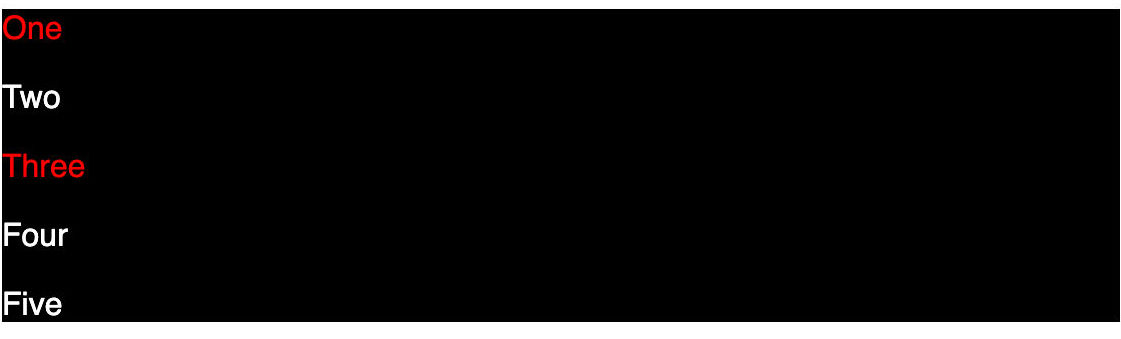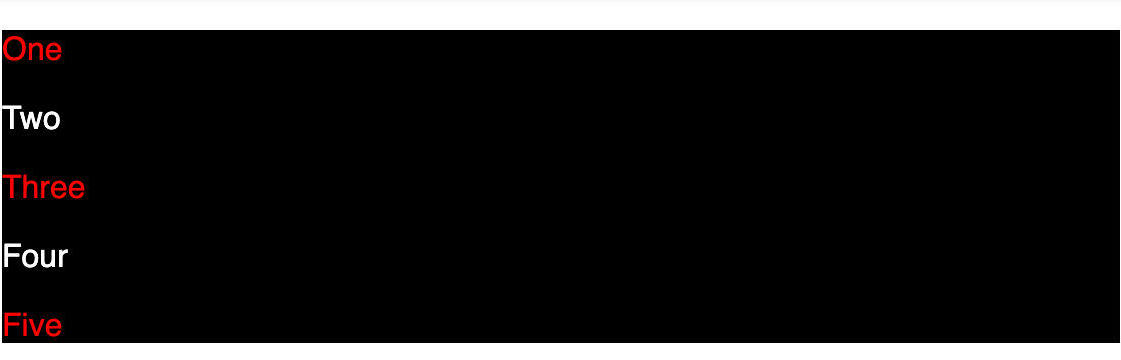# Understanding Nth-Child Selectors in CSS

Saturday, February 18, 2023

The `:nth-child()` CSS pseudo-class matches elements based on their position among a group of siblings i.e. let us say a div has five children where 3 are `p` tags and 2 are `span` tags. If we write the following HTML and CSS --

``````<div>
<p>One</p>
<span>Two</span>
<p>Three</p>
<p>Four</p>
<span>Five</span>
</div>``````
``````div {
background: black;
color: white;
}

div p:nth-child(2n+1) {
color: red;
}``````

It highlights `One` and `Three` only.## Now, why is that?

For this, you need to mainly understand the functional notation syntax of the `:nth-child` selector i.e. `:nth-child(An+B)`. Here

• `A` is the step size
• `B` is the offset
• `n` is the non-negative integer, starting from `0`.

In our example, `:nth-child(2n+1)` where `A=2`, `B=1`, and `n can go from 0,1,2..` to `Infinity`.

``````:nth-child(2(0)+1) => :nth-child(0 + 1) => :nth-child(1)
:nth-child(2(1)+1) => :nth-child(2 + 1) => :nth-child(3)
:nth-child(2(2)+1) => :nth-child(4 + 1) => :nth-child(5)
...``````

## Element Indexing

In our CS classes or our dev work, we are used to having 0-based indices. However, in the case of counting the elements, 1-based indexing is used i.e.

``````<div>
<p>One</p> // 1
<span>Two</span> // 2
<p>Three</p> // 3
<p>Four</p> // 4
<span>Five</span> // 5
</div>``````

The `n` starts from `0` but elements counting starts from `1`. Hence, it matches element `One` and `Three`.

## Why is Five not highlighted in red?

For `:nth-child` selector, the child count includes children of any element type; but it is considered a match only if the element at that child position is of the specified element type. Hence, even though `:nth-child(2n+1)` produces `5` when `n=2` and we have a fifth element, it is highlighted because it is of type `span`. Our condition to match the element must be at the right position and right type.

If we change the fifth element to type `p` then it would be highlighted.

``````<div>
<p>One</p> // 1
<span>Two</span> // 2
<p>Three</p> // 3
<p>Four</p> // 4
<p>Five</p> // 5
</div>``````